# Visualizing complex points of elliptic curves

Date started: September 2019
Leads: Sebastian Bozlee, Silviana Violet Amethyst

###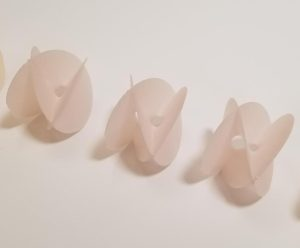Abstract

An elliptic curve over the complex numbers can be described as the set of pairs of complex numbers$(x, y)$ satisfying an equation in the form$y^2 = x^3 + ax + b$, plus a single “point at infinity.” Most pictures of elliptic curves are of only the real solutions$(x, y)$ in$\mathbb{R}^2$. The additional complex solutions$(x, y)$ in$\mathbb{C}^2$ are usually not drawn since$\mathbb{C}^2$ is a real four-dimensional space. It is known that all elliptic curves are topologically torii, but it is difficult to see that this is the case using only the real points. The goal of this project is to visualize these other points, as embedded in$\mathbb{C}^2$, using orthogonal projections of the curve from$\mathbb{C}^2 \cong \mathbb{R}^4$ to$\mathbb{R}^3$.

### Media

We generated four-dimensional computer models of the curve$y^2 = x^3 - x$ using Silviana’s bertini_real software. Here is the result of projecting away the imaginary component of$x$, rendered in Blender:

We then printed a series of models of the curves$y^2 = x(x+1)(x-a)$ with $a$ varying from 1 to 0. This is a degeneration of an elliptic curve to a nodal curve – we see how such curves are limit points of the moduli space of elliptic curves,$\overline{\mathcal{M}}_{1,1}$.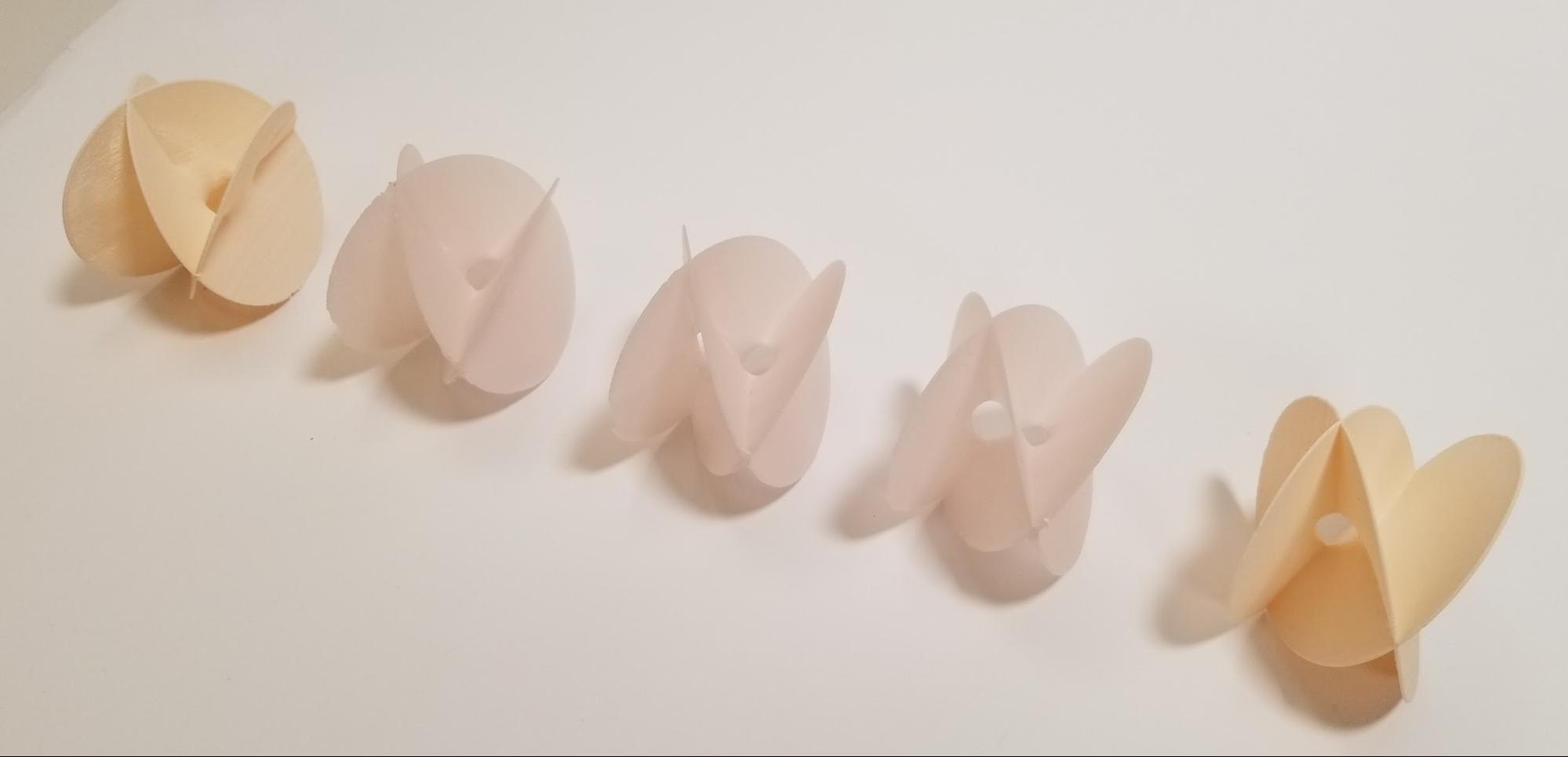We also printed a larger model with the real part emphasized: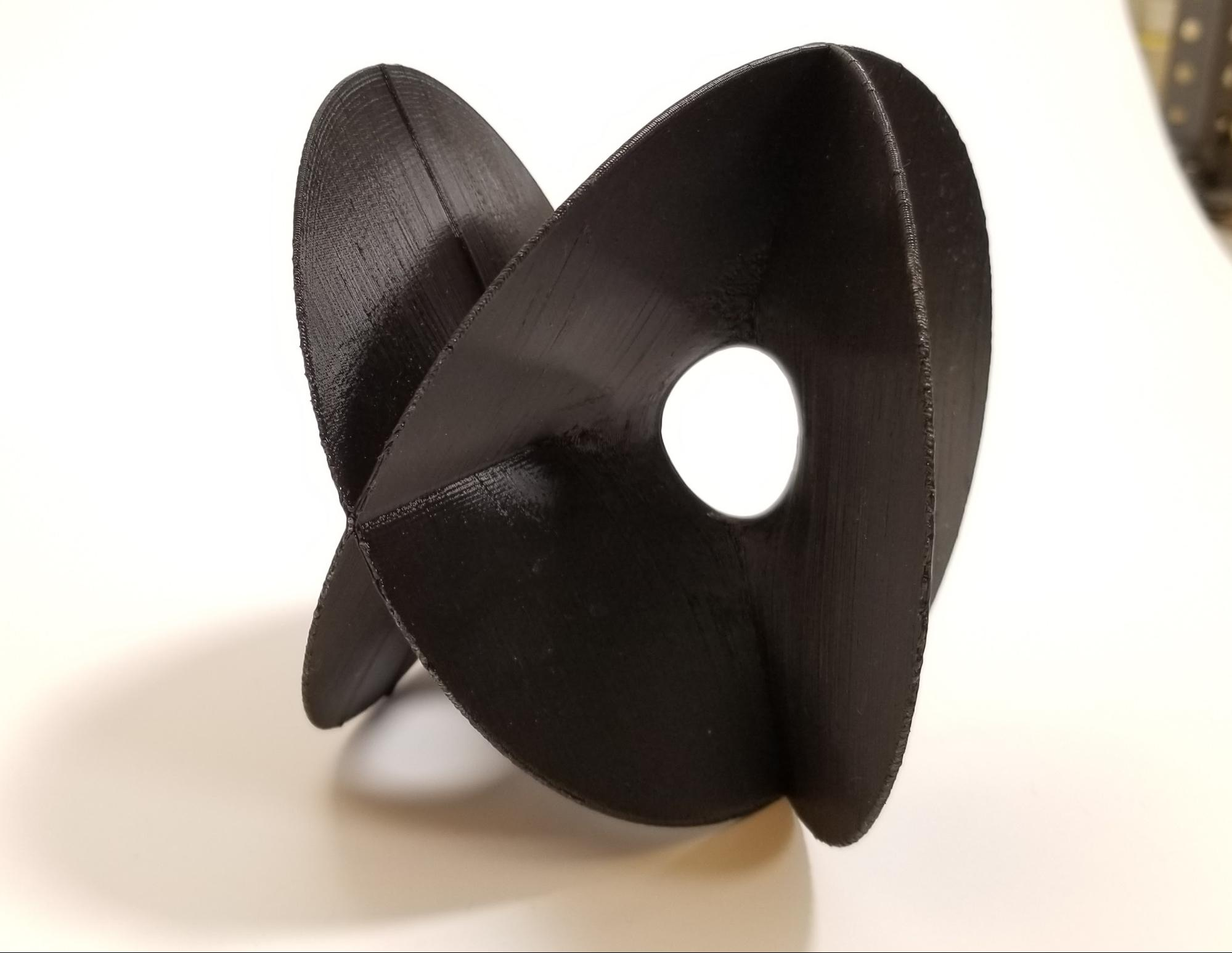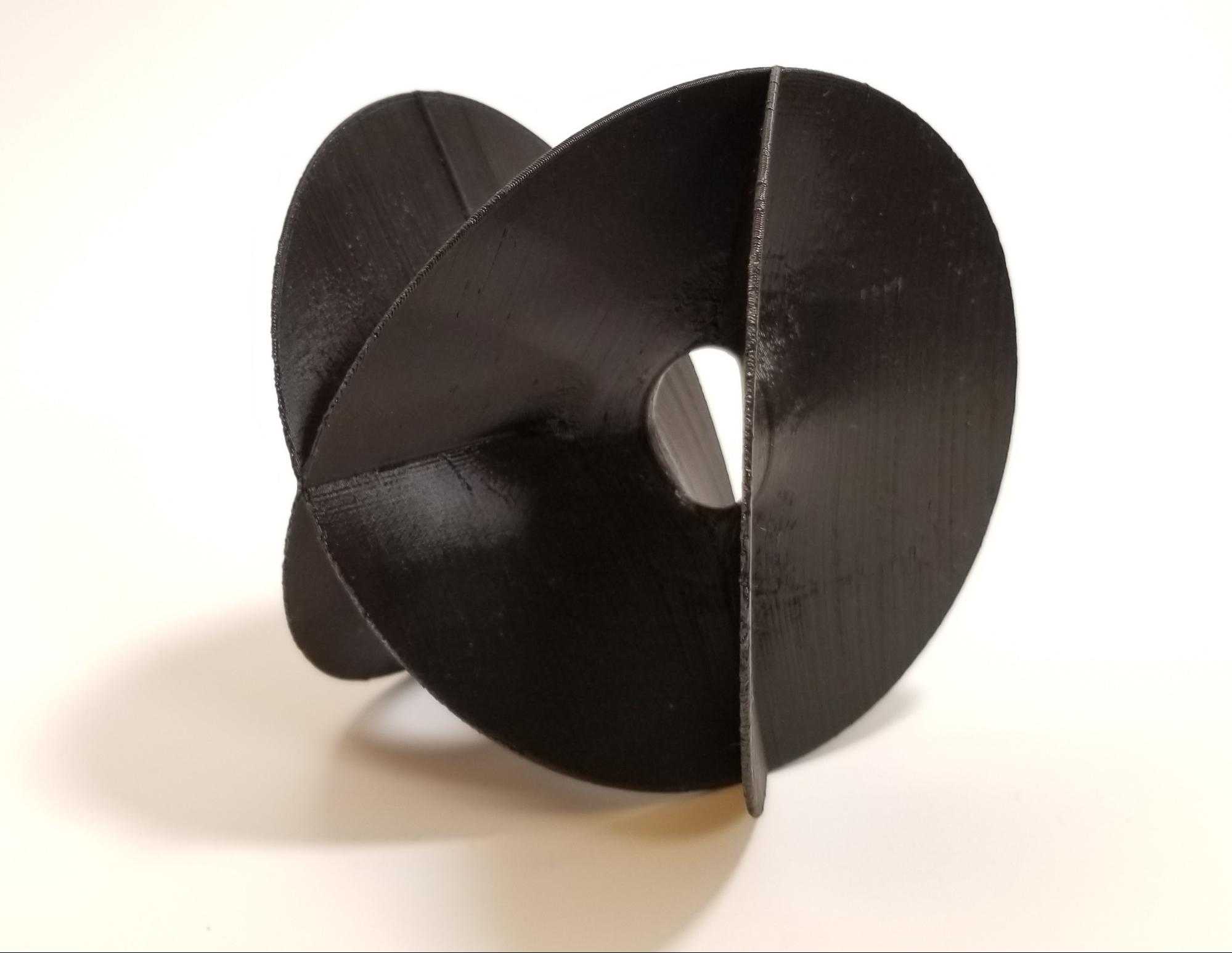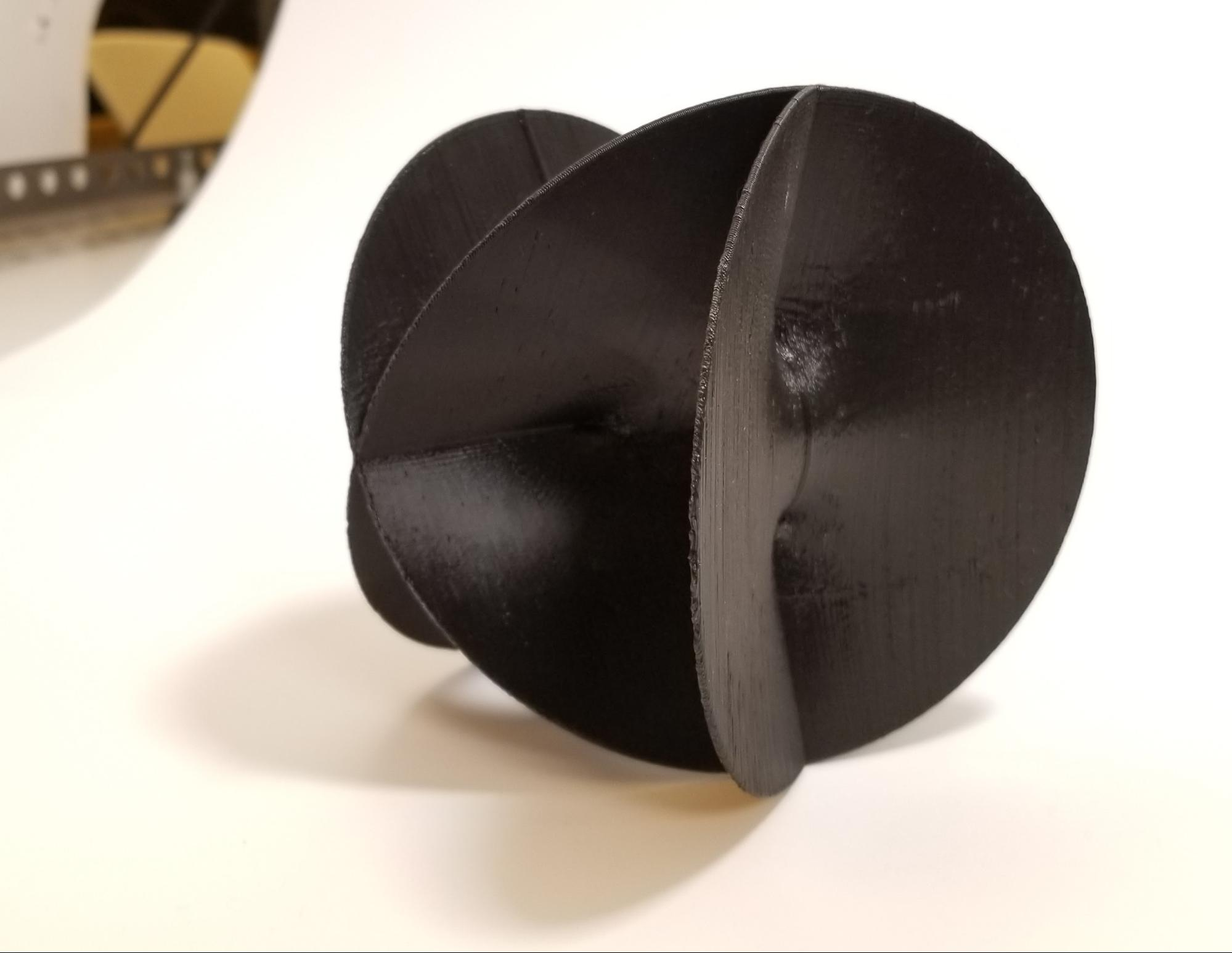Some white paint makes the real part more visible: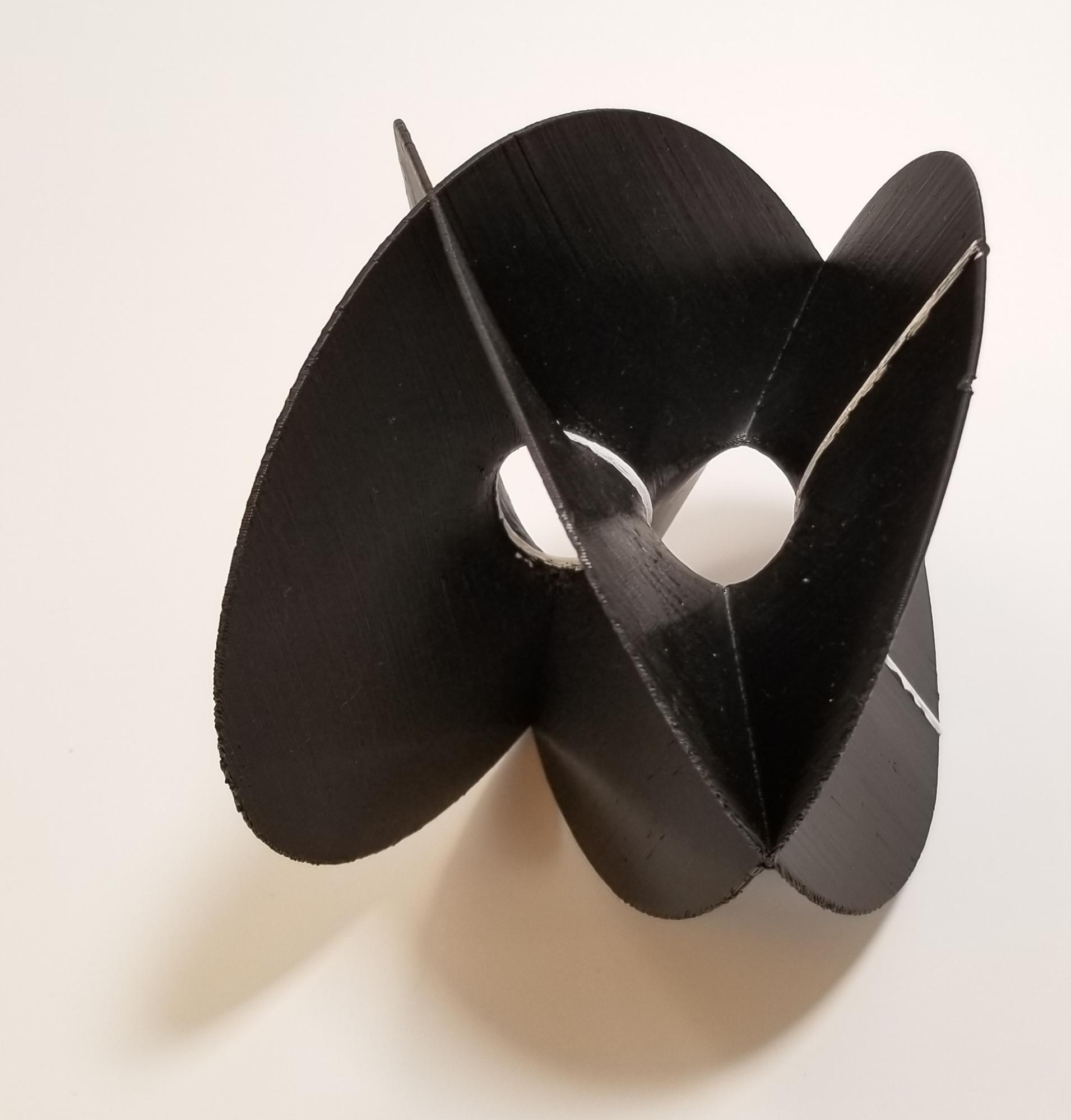If you allow yourself to unfold the knotted surface, you can see the torus: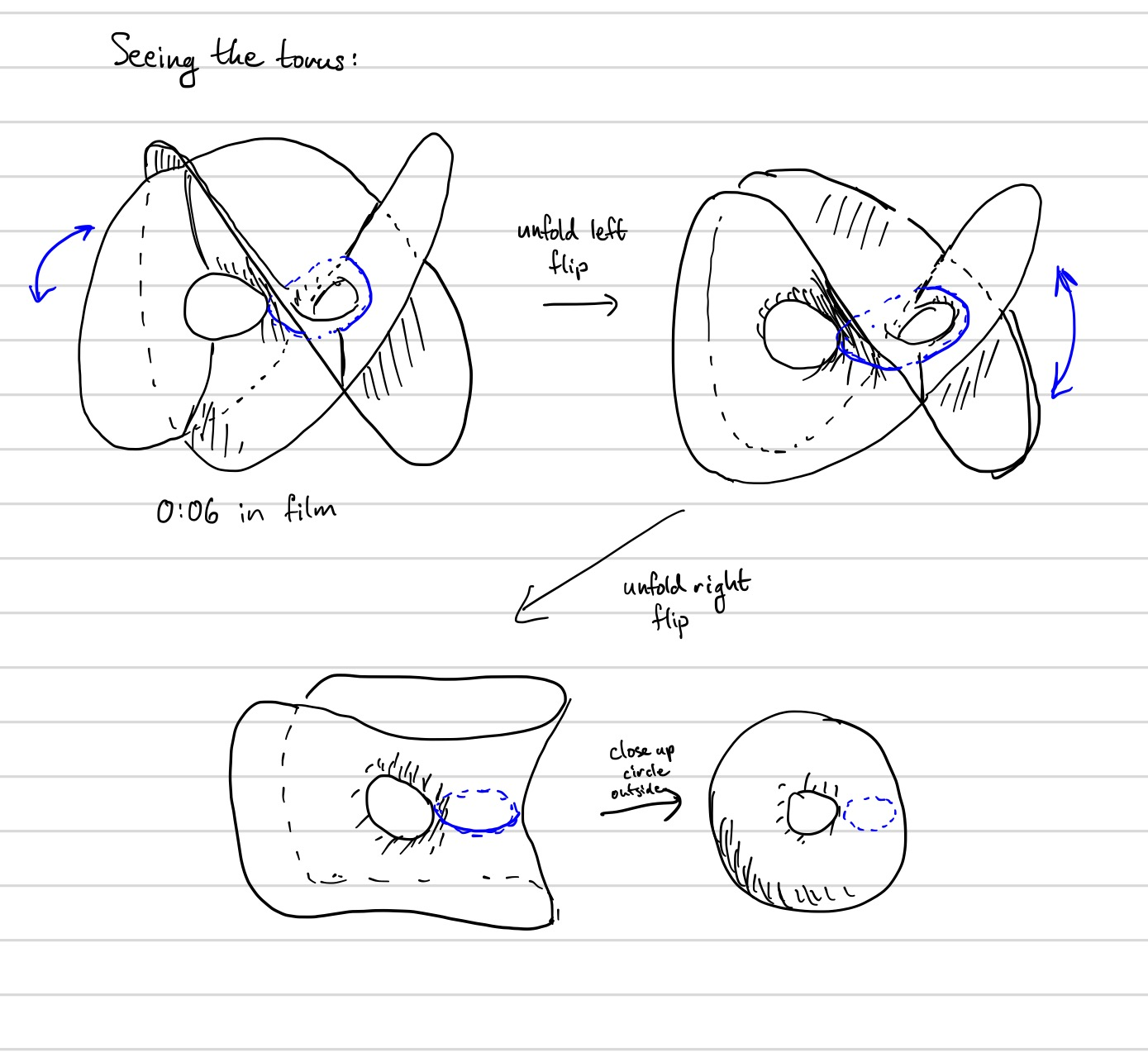Sebastian also wrote software for viewing an elliptic curve in VR, where the user can rotate the object in four dimensions and explore the space of possible projections. In this video we examine some views of the same elliptic curve considered above:

Other elliptic curves are embedded in a different-feeling way: